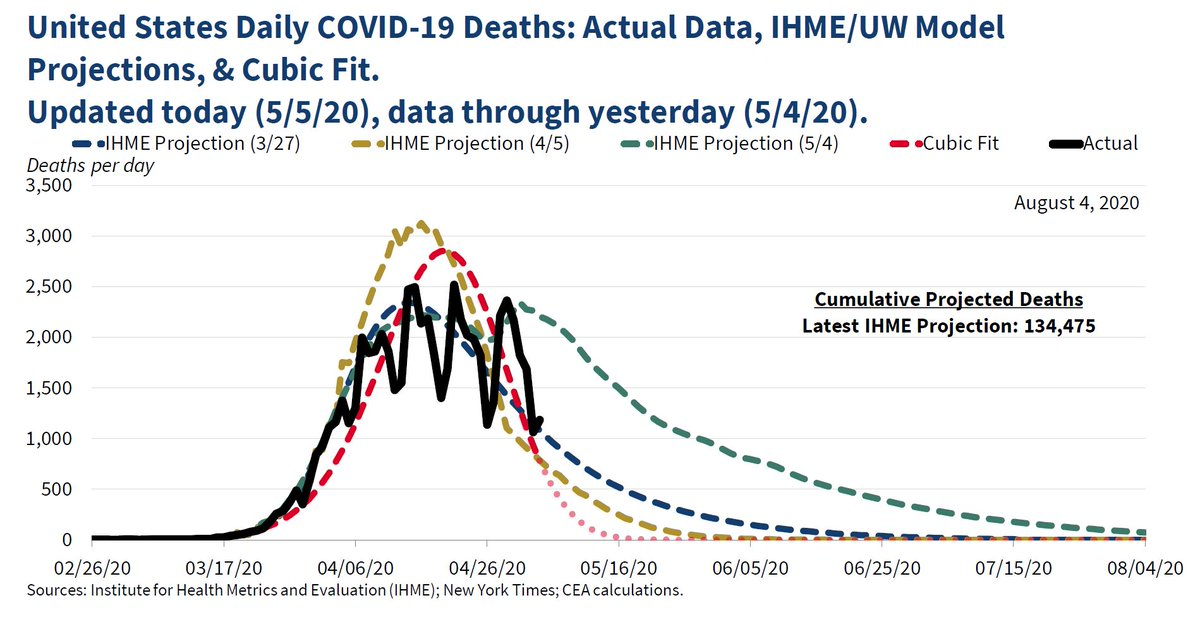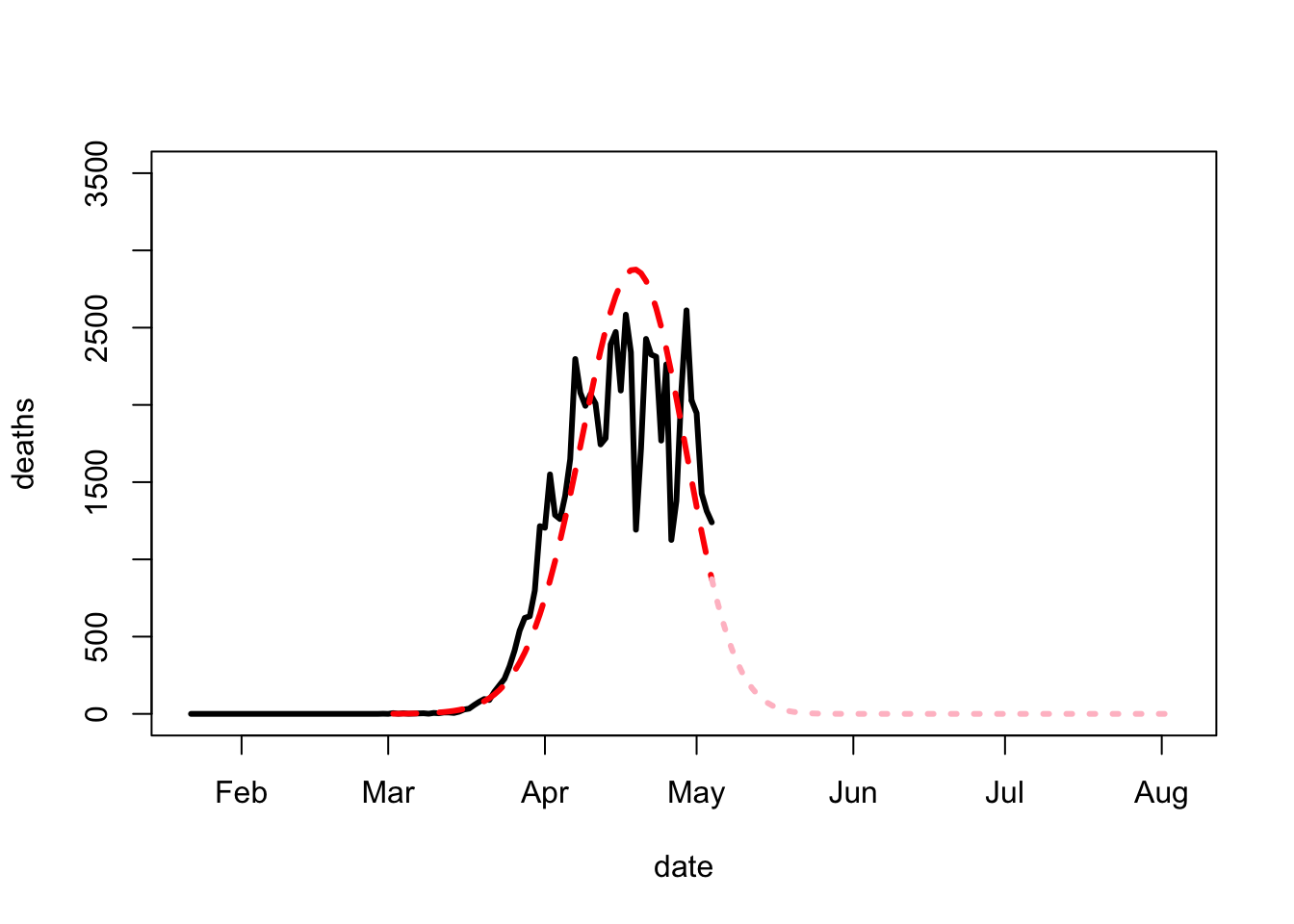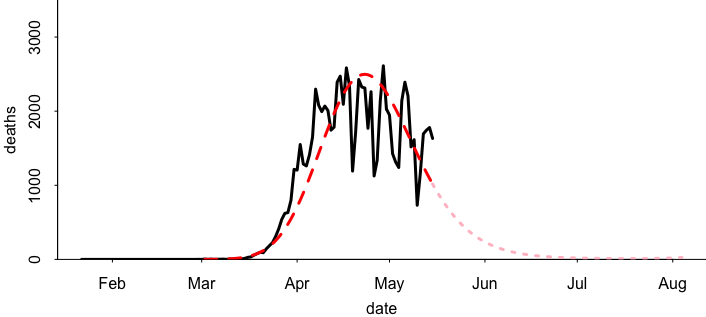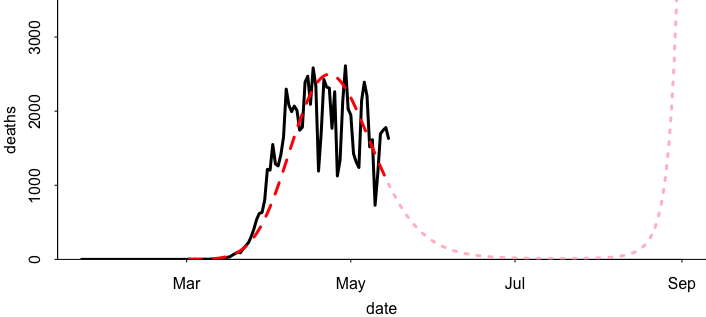# What a difference a month makes (polynomial extrapolation edition)

Someone pointed me to this post from Cosma Shalizi conveniently using R to reproduce the famous graph endorsed by public policy professor and A/Chairman @WhiteHouseCEA.

Here’s the original graph that caused all that annoyance:Here’s Cosma’s reproduction in R (retro-Cosma is using base graphics!), fitting a third-degree polynomial on the logarithms of the death counts:Cosma’s data are slightly different from those in the government graph, but they give the same statistical story.

Its been a couple weeks so I’ll redo the graph running Cosma’s code on current data (making a few changes in display choices):Hey, a cubic still fits the data! Well, not really. According to that earlier graph, the number of new deaths should be approximately zero by now. What happened is that the cubic has shifted, now that we’ve included new data in the fit.

Anyway, here’s my real question.

Cosma is using the same x-axis as the U.S. government was using, going until 4 Aug 2020. But where did 4 Aug come from? That’s kind of a weird date to use as an endpoint. Why, not, say, go until 1 Sept?

Cosma provided code, so it’s trivial to extend the graph to the end of the month, and here’s what we get:Whoa! What happened?

But, yes, of course! A third-degree polynomial doesn’t just go up, then down. It goes up, then down, then up. Here’s the fitted polynomial in question:

```                            coef.est coef.se
(Intercept)                   5.85     0.06
poly(weeks_since_apr_1, 3)1  17.45     0.54
poly(weeks_since_apr_1, 3)2 -10.94     0.54
poly(weeks_since_apr_1, 3)3   1.20     0.54
---
n = 75, k = 4
residual sd = 0.54, R-Squared = 0.95
```

The coefficient of x^3 is positive, so indeed the function has to blow up to infinity once x is big enough. (It blows up to negative infinity for sufficiently low values of x, but since we’re exponentiating to get the prediction back on the original scale, that just sends the fitted curve to zero.)

When I went back and fit the third-degree model just to the data before 5 May, I got this:

```                            coef.est coef.se
(Intercept)                  5.57     0.07
poly(weeks_since_apr_1, 3)1 17.97     0.52
poly(weeks_since_apr_1, 3)2 -8.54     0.52
poly(weeks_since_apr_1, 3)3 -0.97     0.52
---
n = 63, k = 4
residual sd = 0.52, R-Squared = 0.96
```

Now the highest-degree coefficient estimate is negative, so the curve will continue declining to 0 as x increases. It would retrospectively blow up for low enough values of x, but this is not a problem as we’re only going forward in time with our forecasts.